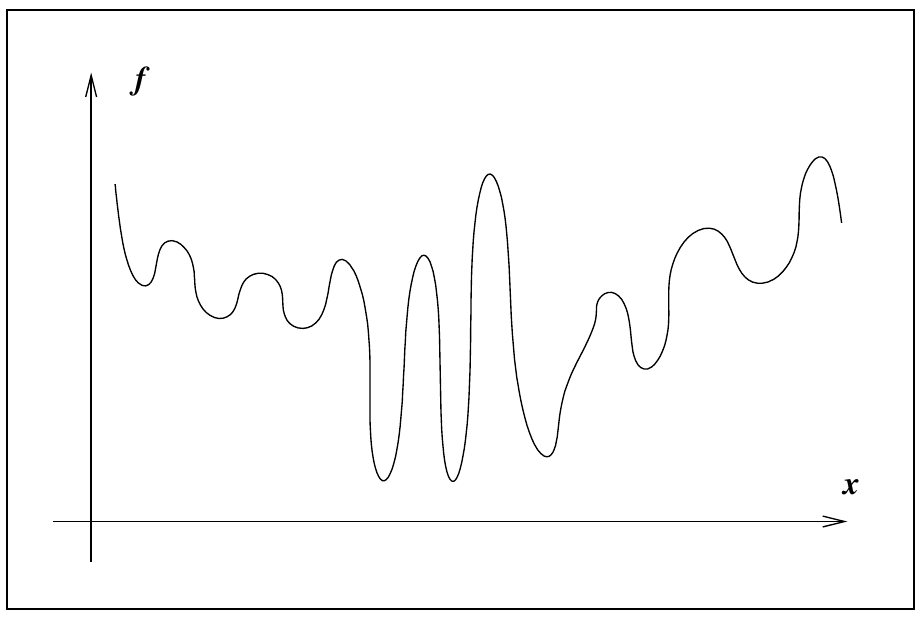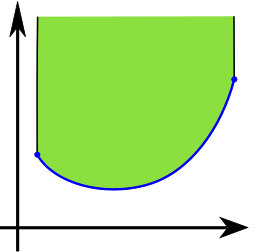# Unconstrained optimization part I

## Instructions:

Use the left and right arrow keys to navigate the presentation forward and backward respectively. You can also use the arrows at the bottom right of the screen to navigate with a mouse.

FAIR USE ACT DISCLAIMER:
This site is for educational purposes only. This website may contain copyrighted material, the use of which has not been specifically authorized by the copyright holders. The material is made available on this website as a way to advance teaching, and copyright-protected materials are used to the extent necessary to make this class function in a distance learning environment. The Fair Use Copyright Disclaimer is under section 107 of the Copyright Act of 1976, allowance is made for “fair use” for purposes such as criticism, comment, news reporting, teaching, scholarship, education and research.

## Outline

• The following topics will be covered in this lecture:
• Concepts in optimization
• Local versus global optimum
• Convexity
• Gradient descent versus Newton descent

## Concepts in optimization

• The maximization and minimization of functions, or optimization problems, contain two components:

1. an objective function $$f(\mathbf{x})$$; and
2. constraints $$g(\mathbf{x})$$.
• E.g., we may wish to optimize factory output $$f(x)$$ as a function of hours $$x$$ in a week, with a measure of our active machine-hours $$g(x)$$ not exceeding a pre-specified limitation $$g(x)\leq C$$.

• Optimization problems can thus be classified into two categories:

• If there are constraints $$g(\mathbf{x})$$ affiliated with the objective function $$f(\mathbf{x})$$, then it is a constrained optimization problem, otherwise, it is a unconstrained optimization problem.
• We will focus on unconstrained optimization as often arises in MLE; this is formulated as the following problem,

\begin{align} f: \mathbb{R}^n &\rightarrow \mathbb{R}\\ \mathbf{x} &\rightarrow f(\mathbf{x})\\ f(\mathbf{x}^\ast) &= \mathrm{max}_{\mathbf{x} \in \mathcal{D}} f \end{align}

• We note that the above problem is equivalent to a minimization problem by a subsitution of $$\tilde{f} = -f$$, i.e.,

\begin{align} \tilde{f}: \mathbb{R}^n &\rightarrow \mathbb{R}\\ \mathbf{x} &\rightarrow -f(\mathbf{x})\\ f(\mathbf{x}^\ast) &= \mathrm{max}_{\mathbf{x} \in \mathcal{D}} \tilde{f} = \mathrm{min}_{\mathbf{x}\in \mathcal{D}} f \end{align}

### Concepts in optimization

• Because these problems are equivalent, we focus on the minimization of functions at the moment as they are traditionally phrased in optimization literature.
• The same techniques will apply for maximization by a simple change of variables.
• We will need to identify a few key concepts: global and local minimizers.
• Suppose we are trying to minimize an objective function $$f$$.
• We would ideally find a global minimizer of $$f$$, a point where the function attains its least value over all possible values under consideration:
A point $$\mathbf{x}^\ast$$ is a global minimizer if $$f(\mathbf{x}^\ast) \leq f(\mathbf{x})$$ for all other possible $$\mathbf{x}$$ in the domain of consideration $$D\subset \mathbb{R}^n$$.
• A global minimizer can be difficult to find, because our knowledge of $$f$$ is usually only local;Courtesy of: J. Nocedal and S. Wright. Numerical optimization. Springer Science & Business Media, 2006.

• i.e., we only can approximate the behavior of the function $$f$$ within small perturbations $$\boldsymbol{\delta}_{x}$$ of values $$\mathbf{x}$$ where we already know $$f(\mathbf{x})$$.
• Since our algorithm hopefully does not need to compute $$f$$ over many points, we usually do not have a good picture of the overall shape of $$f$$,
• generally, we can never be sure that the function does not take a sharp dip in some region that has not been sampled by the algorithm.

### Concepts in optimization

• Most algorithms are able to find only a local minimizer, which is a point that achieves the smallest value of $$f$$ in its neighborhood, i.e.,
Let $$\mathcal{N}\subset \mathcal{D} \subset\mathbb{R}^n$$ be a neighborhood of the point $$\mathbf{x}^\ast$$ in the domain of consideration. We say $$\mathbf{x}^\ast$$ is a local minimizer in the neighborhood of $$\mathcal{N}$$ if \begin{align} f(\mathbf{x}^\ast) \leq f(\mathbf{x}) & & \text{ for each other }\mathbf{x}\in \mathcal{N} \end{align}
• For finding a local minimizer, the main tools will be derived directly from the second order approximation of the objective function $$f$$, defined by

\begin{align} f(\mathbf{x}_1) \approx f(\mathbf{x}_0) + \left(\nabla f(\mathbf{x}_0)\right)^\mathrm{T} \boldsymbol{\delta}_{x_1}+\frac{1}{2} \boldsymbol{\delta}_{x_1}^\mathrm{T}\mathbf{H}_f (\mathbf{x}_0) \boldsymbol{\delta}_{x_1} \end{align}

• We will consider how this is related to the notion of convexity as follows.

### ConvexityCourtesy of: Oleg Alexandrov. Public domain, via Wikimedia Commons.

• Throughout mathematics, the notion of convexity is a powerful tool, often used in optimization.
• Particularly, a function is convex if and only if the region above its graph is a convex set.
• The convexity of the full epigraph set means that the function attains a global minimum over its entire domain.
• In non-convex functions, we can have regions that are also locally convex in the graph of the function.
• For such regions, we can find local minimizers as defined in the last slide.
• In a single variable, this is phrased in terms of the second derivative test, i.e.,
For the function of one variable $$f(x)$$ we say that $$x^\ast$$ is a local minimizer if $$f'(x^\ast)=0$$ and $$f''(x^\ast)> 0$$.
• There is a direct analogy for a function of multiple variables, but this need to be rephrased slightly.

### The second derivative test with the Hessian

• For a real-valued function of multiple variables, $f:\mathbb{R}^n \rightarrow \mathbb{R},$ we will instead phrase the second derivative test in terms of the Hessian of $$f$$, \begin{align} \mathbf{H}_{f} = \begin{pmatrix} \partial_{x_1}^2 f & \cdots & \partial_{x_1}\partial_{x_n}f \\ \vdots &\ddots & \vdots \\ \partial_{x_n}\partial_{x_1} f & \cdots & \partial_{x_n}^2 f \end{pmatrix} \end{align}
• Particularly, the spectral theorem says the Hessian has an eigen decomposition such that by a change of coordinates, $$\mathbf{H}_f$$ will be diagonal.